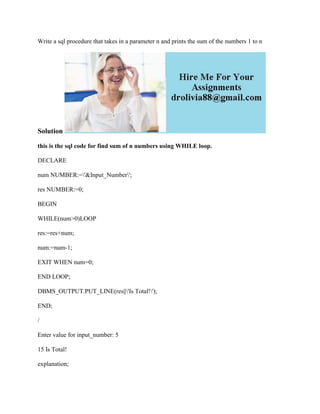Ce diaporama a bien été signalé.
Le téléchargement de votre SlideShare est en cours. ×

# Write a sql procedure that takes in a parameter n and prints the sum o.docx

Publicité
Publicité
Publicité
Publicité
Publicité
Publicité
Publicité
Publicité
Publicité
Publicité
PublicitéChargement dans…3
×

## Consultez-les par la suite

1 sur 2 Publicité

# Write a sql procedure that takes in a parameter n and prints the sum o.docx

Write a sql procedure that takes in a parameter n and prints the sum of the numbers 1 to n
Solution
this is the sql code for find sum of n numbers using WHILE loop.
DECLARE
num NUMBER:=\'&Input_Number\';
res NUMBER:=0;
BEGIN
WHILE(num>0)LOOP
res:=res+num;
num:=num-1;
EXIT WHEN num=0;
END LOOP;
DBMS_OUTPUT.PUT_LINE(res||\'Is Total!\');
END;
/
Enter value for input_number: 5
15 Is Total!
explanation;
first i have declared two variable num and res . frist variable num is for upto how many numbers we are going to calaculate the sum and second variable resis for to store the result that is the running total of series of values from 1 to n numbers . i have calaculated using WHILE LOOP. after calaculating i have printed the sum
.

Write a sql procedure that takes in a parameter n and prints the sum of the numbers 1 to n
Solution
this is the sql code for find sum of n numbers using WHILE loop.
DECLARE
num NUMBER:=\'&Input_Number\';
res NUMBER:=0;
BEGIN
WHILE(num>0)LOOP
res:=res+num;
num:=num-1;
EXIT WHEN num=0;
END LOOP;
DBMS_OUTPUT.PUT_LINE(res||\'Is Total!\');
END;
/
Enter value for input_number: 5
15 Is Total!
explanation;
first i have declared two variable num and res . frist variable num is for upto how many numbers we are going to calaculate the sum and second variable resis for to store the result that is the running total of series of values from 1 to n numbers . i have calaculated using WHILE LOOP. after calaculating i have printed the sum
.

Publicité
Publicité

### Write a sql procedure that takes in a parameter n and prints the sum o.docx

1. 1. Write a sql procedure that takes in a parameter n and prints the sum of the numbers 1 to n Solution this is the sql code for find sum of n numbers using WHILE loop. DECLARE num NUMBER:='&Input_Number'; res NUMBER:=0; BEGIN WHILE(num>0)LOOP res:=res+num; num:=num-1; EXIT WHEN num=0; END LOOP; DBMS_OUTPUT.PUT_LINE(res||'Is Total!'); END; / Enter value for input_number: 5 15 Is Total! explanation;
2. 2. first i have declared two variable num and res . frist variable num is for upto how many numbers we are going to calaculate the sum and second variable resis for to store the result that is the running total of series of values from 1 to n numbers . i have calaculated using WHILE LOOP. after calaculating i have printed the sum